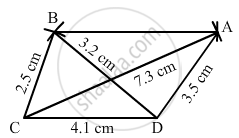Share

# Construct a Quadrilateral Abcd Given Ad = 3.5 Cm, Bc = 2.5 Cm, Cd = 4.1 Cm, Ac = 7.3 Cm And Bd = 3.2 Cm. - Mathematics

Course
ConceptConstructing a Quadrilateral When Two Diagonals and Three Sides Are Given

#### Question

Construct a quadrilateral ABCD given AD = 3.5 cm, BC = 2.5 cm, CD = 4.1 cm, AC = 7.3 cm and BD = 3.2 cm.

#### Solution

Steps of construction:

Step I: Draw CD = 4 . 1 cm .

Step II: With C as the centre and radius 7 . 3 cm, draw an arc .

Step III : With D as the centre and radius 3 . 5 cm, draw an arc to intersect the arc drawn in Step II at A .

Step IV: With D as the centre and radius 3 . 2 cm, draw an arc on the other side of AC .

Step V : With C as the centre and radius 2 . 5 cm, draw an arc to intersect the arc drawn in Step IV at B .

Step VI: Join BA, DA, BC and BD and AC to obtained the required quadrilateral .Is there an error in this question or solution?

#### APPEARS IN

RD Sharma Solution for Mathematics for Class 8 by R D Sharma (2019-2020 Session) (2017 to Current)
Chapter 18: Practical Geometry (Constructions)
Ex. 18.2 | Q: 4 | Page no. 6
Solution Construct a Quadrilateral Abcd Given Ad = 3.5 Cm, Bc = 2.5 Cm, Cd = 4.1 Cm, Ac = 7.3 Cm And Bd = 3.2 Cm. Concept: Constructing a Quadrilateral - When Two Diagonals and Three Sides Are Given.
S